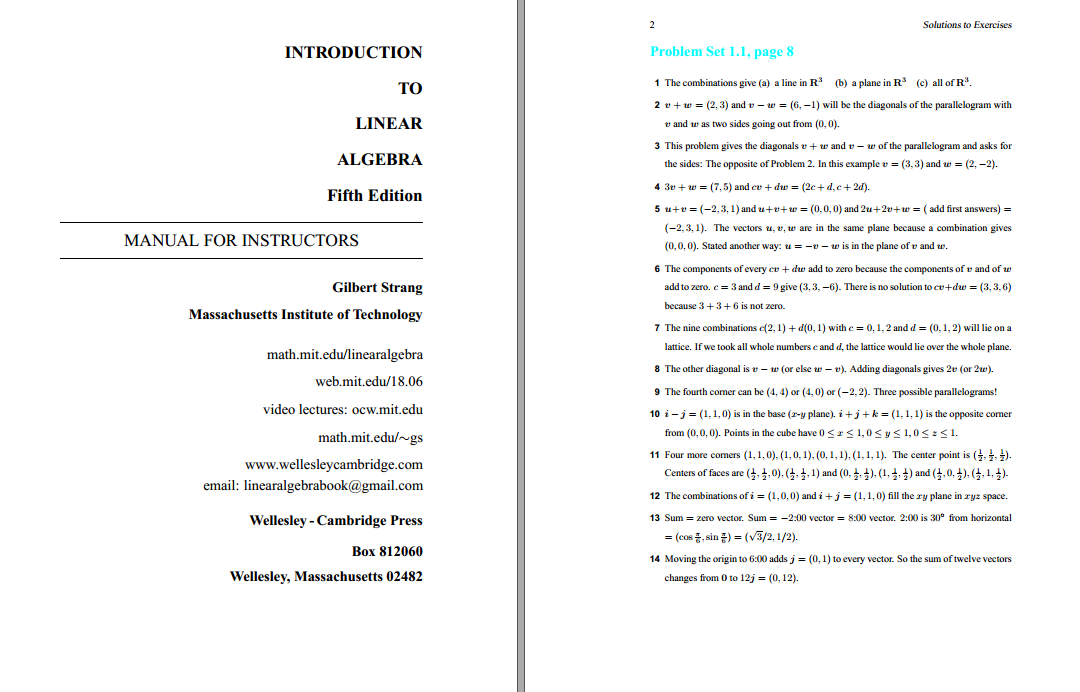# Solution manual for Introduction to Linear Algebra 5th Edition by Gilbert Strang

name：Solution manual for Introduction to Linear Algebra 5th Edition by Gilbert Strang
Edition：5th Edition
author：by Gilbert Strang
ISBN：ISBN-13: 9780980232776
ISBN-10: 0980232775
type：solution manual
format：PDF/zip
All chapter include

Gilbert Strang's textbooks have changed the entire approach to learning linear algebra -- away from abstract vector spaces to specific examples of the four fundamental subspaces: the column space and nullspace of A and A'.
This new fifth edition has become more than a textbook for the basic linear algebra course. That is its first purpose and always will be. The new chapters about applications of the SVD, probability and statistics, and Principal Component Analysis in finance and genetics, make it also a textbook for a second course, plus a resource at work. Linear algebra has become central in modern applied mathematics. This book supports the value of understanding linear algebra.

Introduction to Linear Algebra, Fifth Edition includes challenge problems to complement the review problems that have been highly praised in previous editions. The basic course is followed by eight applications: differential equations in engineering, graphs and networks, statistics, Fourier methods and the FFT, linear programming, computer graphics, cryptography, Principal Component Analysis, and singular values.

##### Cover + PreviewSolution manual for Introduction to Linear Algebra 5th Edition by Gilbert StrangSolution manual for Introduction to Linear Algebra 5th Edition by Gilbert Strang

##### Free Sample
Price：39 points    [1\$ USD=5points]
VIP Price：20% off